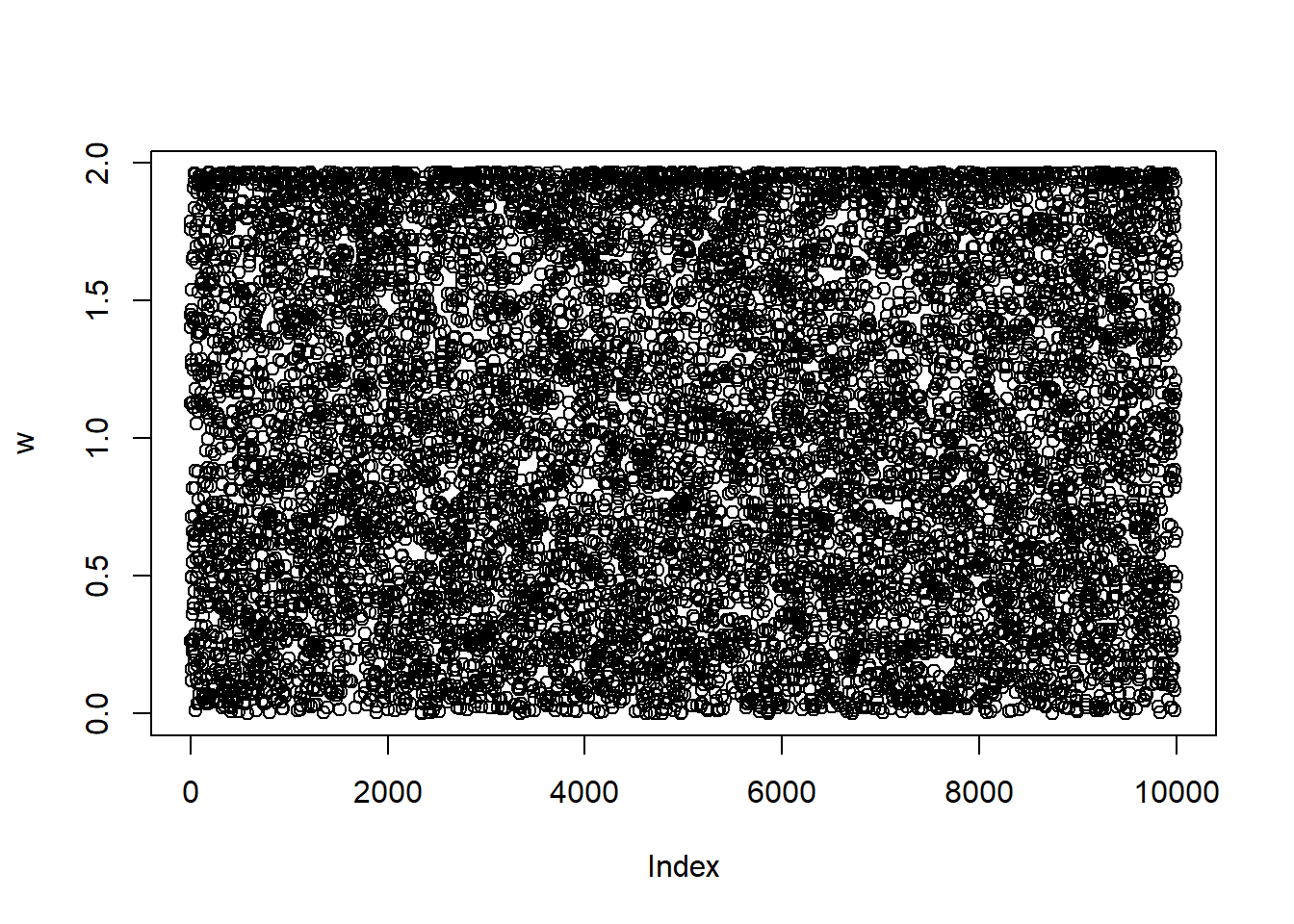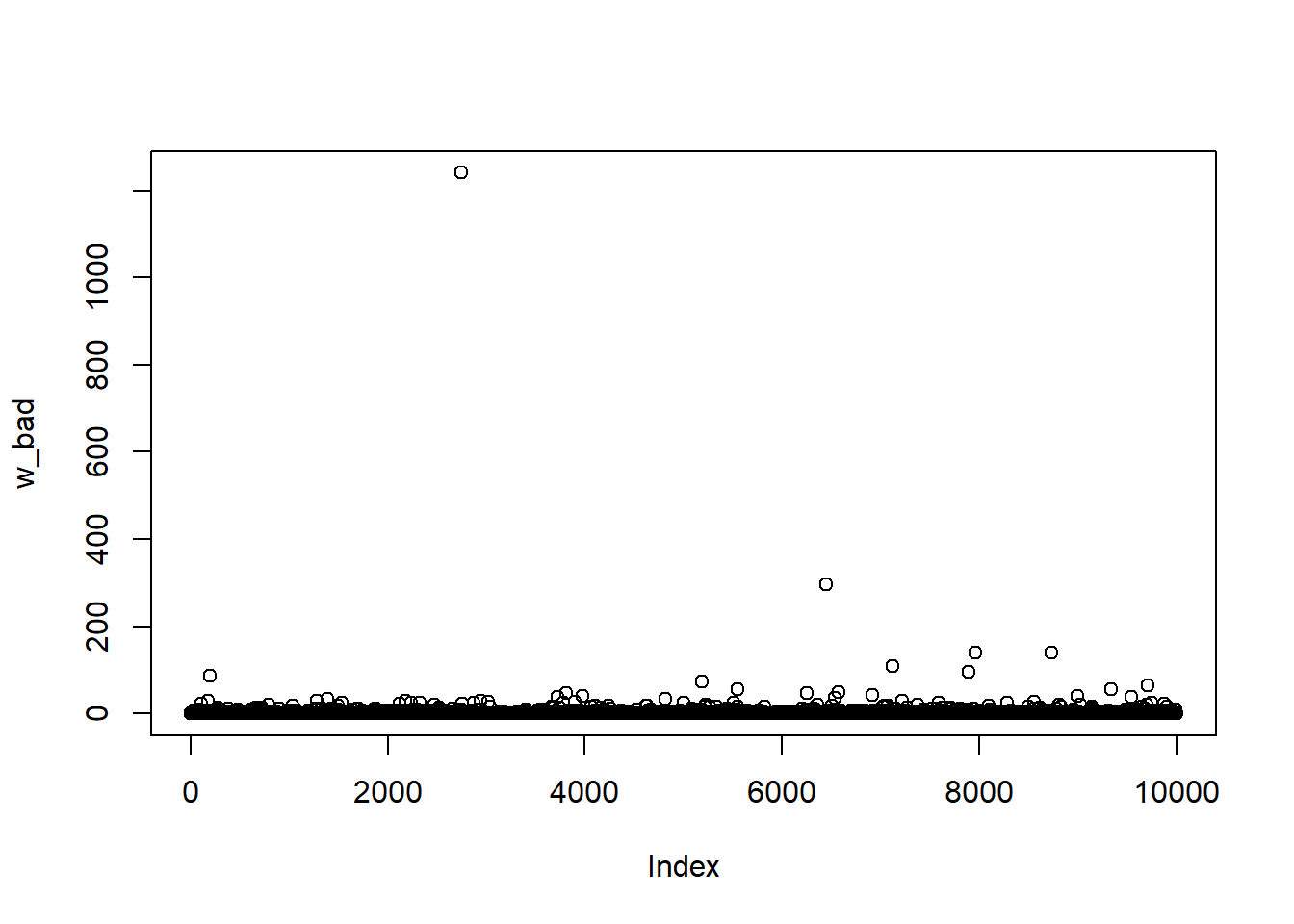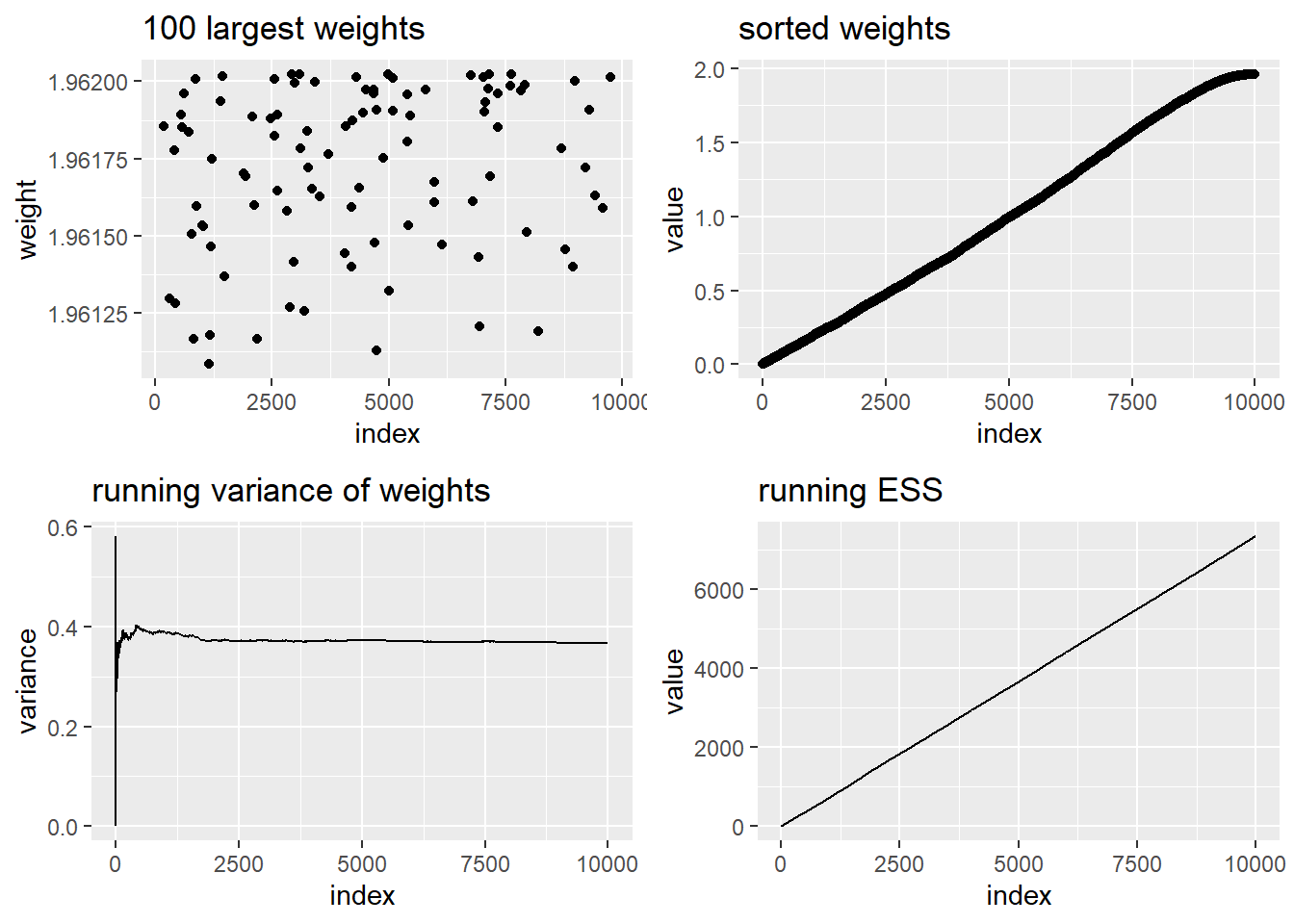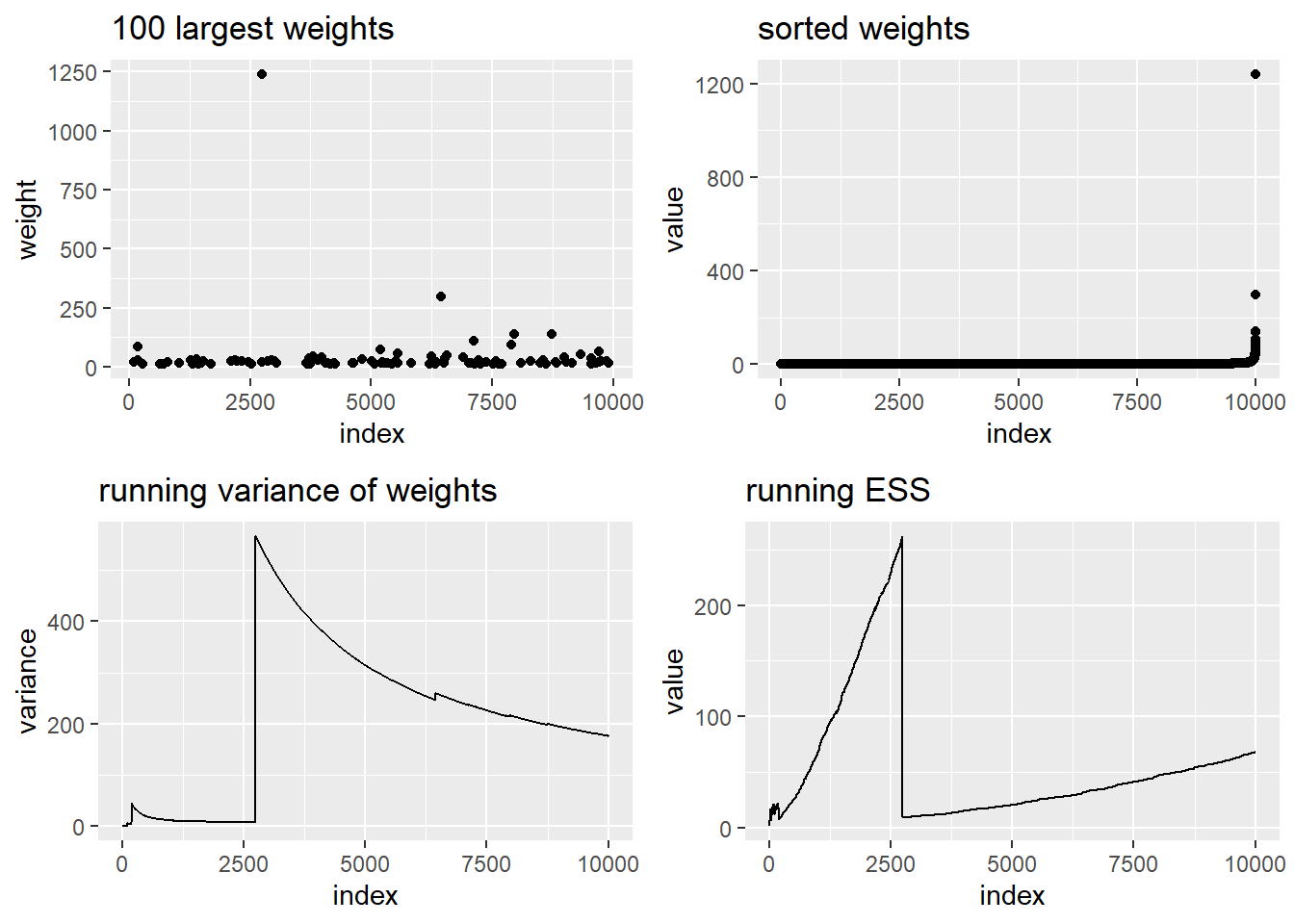# Introduction

diagis is an R package containing functions relating weighted samples obtained for example from importance sampling. The main motivation for developing diagis was to enable easy computation of summary statistics and diagnostics of the weighted IS-MCMC runs provided by bssm package for Bayesian state space modelling. For more broader use, the diagis package provides functions for computing (probability) weighted means, covariances, and quantiles of possibly multivariate samples, the running versions of these, as well as diagnostic plot function weight_plot for graphical diagnostic of weights.

All the mean and covariance functions are written in C++ using Rcpp and RcppArmadillo packages, making these function computationally very efficient even for large samples. The weight diagnostic plot uses ggplot for visually appealing graphics, while gridExtra combines the plots together.

# Illustrations

As an illustration, consider estimating the expected value of Gamma($$\alpha, \beta$$) distribution using importance sampling. The density of Gamma($$\alpha, \beta$$) is $p(x) = \frac{\beta^{\alpha}}{\Gamma(\alpha)} x^{\alpha - 1} \exp(-\beta x).$ We will use another Gamma distribution with parameters $$a$$ and $$b$$ as our proposal distribution $$q(x)$$, so the weights are of form $w(x) = \frac{p(x)}{q(x)} = \frac{\frac{\beta^{\alpha}}{\Gamma(\alpha)} x^{\alpha - 1} \exp(-\beta x)}{\frac{b^{a}}{\Gamma(a)} x^{a - 1} \exp(-b x)} = \frac{\Gamma(a)\beta^{\alpha}}{\Gamma(\alpha)b^a}x^{\alpha - a}\exp(-(\beta -b)x),$ and our importance sampling estimator for $$\theta = \textrm{E}_p(X)$$ is $\hat \theta_n = \frac{1}{n}\sum_{i=1}^n Y_i w(Y_i),$ where $$Y_i, i = 1,\ldots, n$$ are drawn from $$\textrm{Gamma}(a, b)$$. However, in practice we often have access only to unnormalized weights $$w_u(x) = c w(x)$$, which leads to self-normalized importance sampling estimate $\tilde \theta_n = \frac{1}{\sum_{i=1}^n w_u(Y_i)}\sum_{i=1}^n Y_i w_u(Y_i).$

Note that the self-normalized estimate $$\tilde \theta_n$$ is not unbiased, but still consistent. Also, we might want to use self-normalized version even when the computation of weights $$w$$ is possible . Thus the functions in diagis are focused on self-normalized importance sampling as it is more generally applicable approach.

We can in principle choose $$a$$ and $$b$$ arbitrarily, but weights $$w(x)$$ have finite variance only if $$a < \alpha$$ and $$b < \beta$$. As we will soon see, infinite variance of the weights can make the importance sampling unreliable.

Let us first take $$\alpha = 2$$, and $$\beta = 1$$. The expected value is then $$\alpha/\beta = 2$$. For proposal distribution, let us first use $$a = 1$$ and $$b = 0.5$$:

library("diagis")
set.seed(1)
x <- rgamma(10000, 1, 0.75)
w <- dgamma(x, 2, 1) / dgamma(x, 1, 0.75)
plot(w)weighted_mean(x, w)
##  2.012761

Our estimate is fairly close to the theoretical value, and the plot of weights $$w$$ suggest that the distribution of the weights is nearly uniform with upper bound slightly below 2 (actual bound can be computed theoretically based on $$w(x)$$).

Let us now change $$a$$ to 2:

set.seed(1)
x_bad <- rgamma(10000, 1, 2)
plot(w_bad)weighted_mean(x_bad, w_bad)
##  2.313655

Now our importance sampling estimate is cleary off, and the plot of the importance weights show what is going on: There is one huge weight and couple others which will dominate our mean estimator. We can use weight_plot function to further illustrate the differences between our two approaches. First let’s check the results from our good IS case:

weight_plot(w)The function weight_plot draws four figures. The first one shows 100 largest weights, again illustrating that there are no single draws dominating the sample. On the second figure, the weights are sorted and drawn in increasing order. This figure has mixed uses, sometimes it shows interesting phenomena better than for example a histogram (which often needs some tweaking), whereas in other cases its information value is quite small. Here we see that the weights are distributed quite uniformly. The third figure is often the most important; it shows the variance of the weights computed from successive samples \$w_1, , w_t, $$t = 1, \ldots, n$$. If the importance weights have finite variance, this figure should show clear converge towards finite values as is the case here. The final figure shows the effective sample sizes again in a form of running line. The effective sample size (ESS) is defined as $ESS_n = \frac{(\sum_{i=1}^n w_i)^2}{\sum_{i=1}^n w^2_i} = \frac{1}{\sum_{i=1}^n \bar w^2_i},$ where $$\bar w_i = w_i / \sum_{i=1}^n w_i$$. The interpretation of ESS is that our importance sampling corresponds to the case with direct simulation from the target distribution $$p(x)$$ using ESS samples (i.e. larger the ESS is better). Other measures of efficiency are also available in literature, and they might be added to diagis in future.

Now let’s use weight_plot function for our bad IS run:

weight_plot(w_bad)Oops! The figures are pretty self-explanatory, the running statistics look fine at first, but when the we take that one huge weight into account, the variance explodes and at the same time ESS drops significantly as that one $$(x_i, w_i)$$ pair dominates the sums in ESS.

# Weighted quantiles

The diagis package also contains function weighted_quantile for computing weighted quantiles (or perhaps more precisely percentiles, but we call them quantiles as ìn quantile method in base R). As for non-weighted quantiles, there are several ways to compute weighted quantiles, and the one used in diagis is based on the following linear interpolation of the weighted empirical CDF (in nonweighted case, this corresponds to type 4 quantile in quantile). Let $$x_1,\ldots,x_n$$ be an ordered (increasing) vector, $$w_1,\ldots,w_n$$ the corresponding weights with $$\sum_{i=1}^n w_i=1$$, and $$p$$ the target probability for which we want to find the corresponding quantile $$q$$. Now let $$w'_j=\sum_{i=1}^jw_i$$ be the cumulative sum of weights from $$1$$ to $$j$$, and find index $$k$$ so that $$w_{k-1} < p$$ and $$w_k \geq p$$. Then

$q = x_{k-1} + (x_k - x_{k-1})\frac{p - w_{k-1}}{w_{k} - w_{k-1}}.$ In case where $$x$$ can contain several identical values, these elements should be combined (and corresponding weights added together), which is done automatically in weighted_quantile.